# High School Chemistry : Help with Reaction Rate

## Example Questions

### Example Question #1 : Kinetics

Which of the following does NOT speed up a reaction?

Increase the reactant concentrations

Increase the temperature

Explanation:

There are a few things that can speed up the rate of a reaction. You can increase the temperature or reactant concentrations. You can also add a catalyst to the reaction. Increasing temperature will ensure that reactant molecules move more quickly in solution, causing more collisions and faster reactions. Essentially, increasing temperature will increase the energy available to fuel the reaction. Adding a catalyst decreases the activation energy of the reaction, meaning that the reaction requires less work. Increasing reactant concentrations will shift equilibrium toward the products via Le Chatelier's principle, causing the reaction to speed up in that direction.

Slowly pouring the reactant into the solution will not increase the reaction rate, and can potentially slow it down.

### Example Question #1 : Kinetics

Which of the following statements is NOT true concerning catalysts?

They lower the activation energy

They increase both the forward and reverse reaction rates

They result in more products

They are not used up in the reaction

They result in more products

Explanation:

Catalysts and enzymes (biological catalysts) function to increase the rate at which a reaction takes place. They do not affect the amount of products created once at equilibrium. In other words, catalysts will affect kinetics, but not equilibrium. Equilibrium can only be affected by Le Chatelier principles, including temperature change, reactant/product concentration change, or pressure change (for a system involving gases).

Catalysts act by lowering the activation energy of a reaction and stabilizing the transition states. They are not consumed or produced by the reaction, meaning that they are not included in the net reaction equation. Both the forward and reverse reactions will be affected. When the forward reaction is favored by equilibium principles, the catalyst helps make products. When the reverse reaction is favored, the catalyst will still function to help create reactants.

### Example Question #3 : Kinetics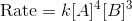If the concentration of each reactant is increased by a factor of two, how much faster will the reaction occur?

128 times faster

8 times faster

76 times faster

16 times faster

246 times faster

128 times faster

Explanation:

We can compare the rates by setting up the following equations: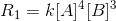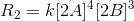We can expand the second equation by distributing the exponent: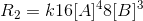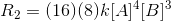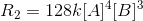We can see that the rate is multiplied by a factor of 128 when the concentration of each reactant is doubled.

### Example Question #541 : High School ChemistryIf the concentration of each reactant is increased by a factor of two, how much faster will the reaction occur?

16 times faster

8 times faster

128 times faster

246 times faster

128 times faster

Explanation:

We can compare the rates by setting up the following equations:We can expand the second equation by distributing the exponent:We can see that the rate is multiplied by a factor of 128 when the concentration of each reactant is doubled.

### Example Question #5 : KineticsIf the concentration of each reactant is increased by a factor of two, how much faster will the reaction occur?

128 times faster

246 times faster

16 times faster

8 times faster

128 times faster

Explanation:

We can compare the rates by setting up the following equations:We can expand the second equation by distributing the exponent:We can see that the rate is multiplied by a factor of 128 when the concentration of each reactant is doubled.

### All High School Chemistry Resources